# Resources tagged with: Calculating with fractions

Filter by: Content type:
Age range:
Challenge level:

There are 63 NRICH Mathematical resources connected to Calculating with fractions, you may find related items under Fractions, Decimals, Percentages, Ratio and Proportion.

Broad Topics > Fractions, Decimals, Percentages, Ratio and Proportion > Calculating with fractions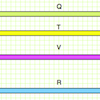### More Fraction Bars

##### Age 7 to 11 Challenge Level:

What fraction of the black bar are the other bars? Have a go at this challenging task!### A4 Fraction Subtraction

##### Age 7 to 11 Challenge Level:

This task offers opportunities to subtract fractions using A4 paper.##### Age 7 to 11 Challenge Level:

Try adding fractions using A4 paper.##### Age 11 to 14 Challenge Level:

Aisha's division and subtraction calculations both gave the same answer! Can you find some more examples?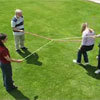### Tangling and Untangling

Twisting and turning with ropes can be encoded mathematically using fractions. Can you find a way to get back to zero?### Jumping

##### Age 7 to 11 Challenge Level:

After training hard, these two children have improved their results. Can you work out the length or height of their first jumps?### Ratios and Dilutions

##### Age 14 to 16 Challenge Level:

Scientists often require solutions which are diluted to a particular concentration. In this problem, you can explore the mathematics of simple dilutions##### Age 11 to 14 Challenge Level:

Can you work out which drink has the stronger flavour?### Diminishing Returns

##### Age 11 to 14 Challenge Level:

How much of the square is coloured blue? How will the pattern continue?### The Greedy Algorithm

##### Age 11 to 14 Challenge Level:

The Egyptians expressed all fractions as the sum of different unit fractions. The Greedy Algorithm might provide us with an efficient way of doing this.### Keep it Simple

##### Age 11 to 14 Challenge Level:

Can all unit fractions be written as the sum of two unit fractions?### More Twisting and Turning

##### Age 11 to 16 Challenge Level:

It would be nice to have a strategy for disentangling any tangled ropes...### Twisting and Turning

##### Age 11 to 14 Challenge Level:

Take a look at the video and try to find a sequence of moves that will untangle the ropes.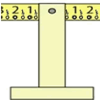### Balance of Halves

##### Age 7 to 11 Challenge Level:

Investigate this balance which is marked in halves. If you had a weight on the left-hand 7, where could you hang two weights on the right to make it balance?### A Chance to Win?

##### Age 11 to 14 Challenge Level:

Imagine you were given the chance to win some money... and imagine you had nothing to lose...### Making Longer, Making Shorter

##### Age 5 to 7 Challenge Level:

Ahmed is making rods using different numbers of cubes. Which rod is twice the length of his first rod?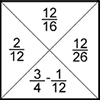### Fractions Jigsaw

##### Age 11 to 14 Challenge Level:

A jigsaw where pieces only go together if the fractions are equivalent.### Harmonic Triangle

##### Age 14 to 16 Challenge Level:

Can you see how to build a harmonic triangle? Can you work out the next two rows?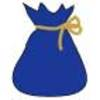### Andy's Marbles

##### Age 7 to 11 Challenge Level:

Andy had a big bag of marbles but unfortunately the bottom of it split and all the marbles spilled out. Use the information to find out how many there were in the bag originally.### Orange Drink

##### Age 7 to 11 Challenge Level:

A 750 ml bottle of concentrated orange squash is enough to make fifteen 250 ml glasses of diluted orange drink. How much water is needed to make 10 litres of this drink?### Ben's Game

##### Age 11 to 14 Challenge Level:

Ben passed a third of his counters to Jack, Jack passed a quarter of his counters to Emma and Emma passed a fifth of her counters to Ben. After this they all had the same number of counters.### Fair Feast

##### Age 5 to 11 Challenge Level:

Here is a picnic that Petros and Michael are going to share equally. Can you tell us what each of them will have?### Peaches Today, Peaches Tomorrow...

##### Age 11 to 14 Challenge Level:

Whenever a monkey has peaches, he always keeps a fraction of them each day, gives the rest away, and then eats one. How long could he make his peaches last for?### Egyptian Fractions

##### Age 11 to 14 Challenge Level:

The Egyptians expressed all fractions as the sum of different unit fractions. Here is a chance to explore how they could have written different fractions.##### Age 11 to 16 Challenge Level:

The items in the shopping basket add and multiply to give the same amount. What could their prices be?### Fractions in a Box

##### Age 7 to 11 Challenge Level:

The discs for this game are kept in a flat square box with a square hole for each. Use the information to find out how many discs of each colour there are in the box.### Blue and White

##### Age 11 to 14 Challenge Level:

Identical squares of side one unit contain some circles shaded blue. In which of the four examples is the shaded area greatest?### Fair Shares?

##### Age 14 to 16 Challenge Level:

A mother wants to share a sum of money by giving each of her children in turn a lump sum plus a fraction of the remainder. How can she do this in order to share the money out equally?### There's a Limit

##### Age 14 to 18 Challenge Level:

Explore the continued fraction: 2+3/(2+3/(2+3/2+...)) What do you notice when successive terms are taken? What happens to the terms if the fraction goes on indefinitely?### A Swiss Sum

##### Age 16 to 18 Challenge Level:

Can you use the given image to say something about the sum of an infinite series?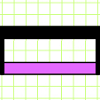### Extending Fraction Bars

##### Age 7 to 11 Challenge Level:

Can you compare these bars with each other and express their lengths as fractions of the black bar?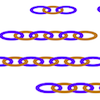##### Age 7 to 11 Challenge Level:

Can you find ways to make twenty-link chains from these smaller chains? This gives opportunities for different approaches.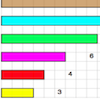### Fraction Lengths

##### Age 7 to 11 Challenge Level:

Can you find combinations of strips of paper which equal the length of the black strip? If the length of the black is 1, how could you write the sum of the strips?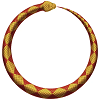### Snake Coils

##### Age 7 to 11 Challenge Level:

This challenge asks you to imagine a snake coiling on itself.### Tray Bake

##### Age 11 to 14 Challenge Level:

My recipe is for 12 cakes - how do I change it if I want to make a different number of cakes?### Reductant Ratios

##### Age 16 to 18 Challenge Level:

What does the empirical formula of this mixture of iron oxides tell you about its consituents?### Countdown Fractions

##### Age 11 to 16 Challenge Level:

Here is a chance to play a fractions version of the classic Countdown Game.### Investigating the Dilution Series

##### Age 14 to 16 Challenge Level:

Which dilutions can you make using only 10ml pipettes?### All Tangled Up

##### Age 14 to 18 Challenge Level:

Can you tangle yourself up and reach any fraction?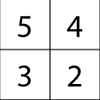### Magic Matrix

##### Age 7 to 11 Challenge Level:

Find out why these matrices are magic. Can you work out how they were made? Can you make your own Magic Matrix?### Once Upon a Time

##### Age 7 to 11 Challenge Level:

Can you work out the height of Baby Bear's chair and whose bed is whose if all the things the three bears have are in the same proportions?### The Harmonic Triangle and Pascal's Triangle

##### Age 16 to 18

The harmonic triangle is built from fractions with unit numerators using a rule very similar to Pascal's triangle.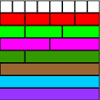##### Age 7 to 11 Challenge Level:

Use the fraction wall to compare the size of these fractions - you'll be amazed how it helps!### Fracmax

##### Age 14 to 16 Challenge Level:

Find the maximum value of 1/p + 1/q + 1/r where this sum is less than 1 and p, q, and r are positive integers.### And So on - and on -and On

##### Age 16 to 18 Challenge Level:

Can you find the value of this function involving algebraic fractions for x=2000?### Hello Again

##### Age 11 to 14 Challenge Level:

Anne completes a circuit around a circular track in 40 seconds. Brenda runs in the opposite direction and meets Anne every 15 seconds. How long does it take Brenda to run around the track?### Smaller and Smaller

##### Age 7 to 14 Challenge Level:

Can you predict, without drawing, what the perimeter of the next shape in this pattern will be if we continue drawing them in the same way?### Hands Together

##### Age 11 to 14 Challenge Level:

Sometime during every hour the minute hand lies directly above the hour hand. At what time between 4 and 5 o'clock does this happen?### Fun Time

##### Age 7 to 11 Challenge Level:

On Saturday, Asha and Kishan's grandad took them to a Theme Park. Use the information to work out how long were they in the theme park.### In the Money

##### Age 7 to 11 Challenge Level:

One quarter of these coins are heads but when I turn over two coins, one third are heads. How many coins are there?